Homework Help Question & Answers

# A sample of sulfur trioxide (SO3) weighs 5100g. How many molecules are contained in this sample?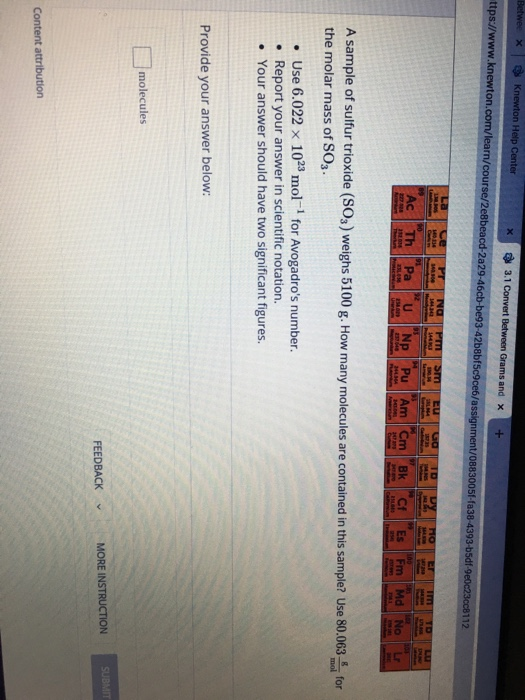A sample of sulfur trioxide (SO3) weighs 5100g. How many molecules are contained in this sample? Use 80.063 the molar mass of SO3.

Use 6.022 x 1023 mol-1 for Avogadro's number.

Report your answer in scientific notation.

Your answer should have two significant figures.

#### Homework Answers

Answer #1 ✔ Recommended Answer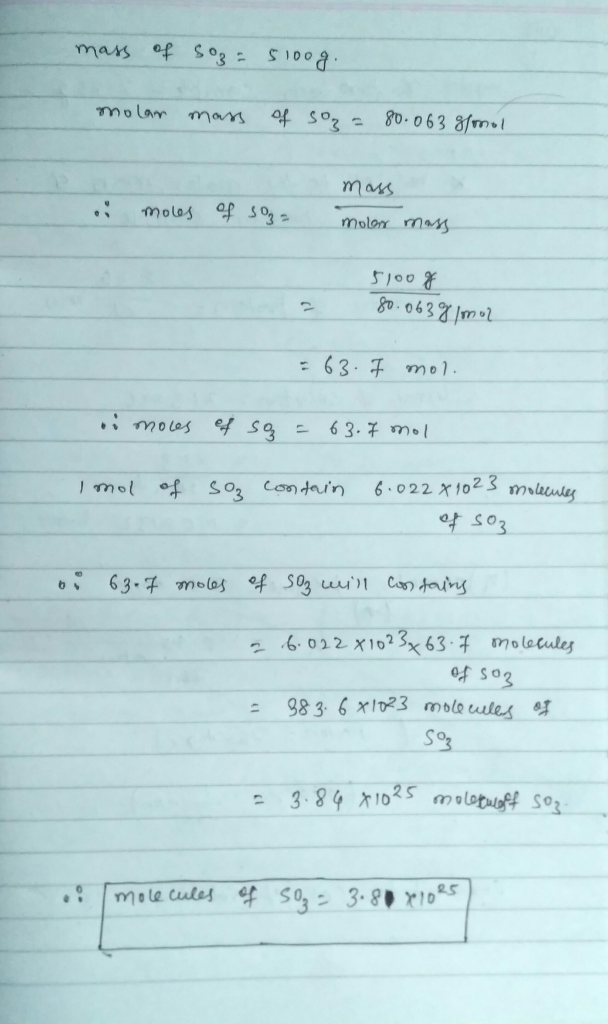Ans-3.8×10^25 molecules of SO3

Add a comment
Know the answer?
Your Answer:

#### Post as a guest

Your Name:

What's your source?

#### Earn Coin

Coins can be redeemed for fabulous gifts.

Similar Homework Help Questions
• ### How many C atoms are present in the sample of C3H8 with mass 4.41 g? Avogadro’s...

How many C atoms are present in the sample of C3H8 with mass 4.41 g? Avogadro’s number is 6.022 × 1023. Answer using scientific notation and to three significant digits.

• ### 1.) Acid rain forms in the upper atmosphere by the reaction of sulfur trioxide with water...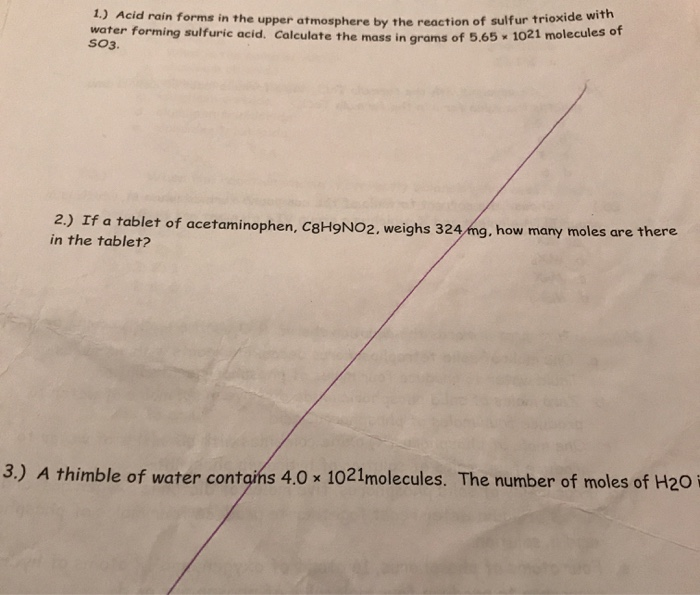1.) Acid rain forms in the upper atmosphere by the reaction of sulfur trioxide with water forming sulfuric acid. Calculate the mass in grams of 5.65 1021 molecules of SO3. 2.) If a tablet of acetaminophen, C8H9NO2, weighs 324 mg, how many moles are there in the tablet? 3.) A thimble of water contains 4.0 x 1021molecules. The number of moles of H20 i

• ### How many grams of Al are in 1.38 * 1023 molecules of Al2O3 if the atomic...

How many grams of Al are in 1.38 * 1023 molecules of Al2O3 if the atomic mass of Al is 27.0 g/mole, O is 16.0 g/mole, and Al2O3 is 102.0 g/mole (the final answer should have the correct number of significant figures)?   Here is my work: (1.38*10^23 molecules) (1mol / 6.022*10^23 molecules) (27.0 gram/ mol)= 6.1873 I counted 3 Sig Fig so I rounded my answer to 6.19.... Another thought was (1.38*10^23 molecules) (54grams /1 mol) (1mol/6.022*10^23) but that answer...

• ### Part A How many sulfur molecules are there in 0.570 mol of octasulfur, Sg? Express your...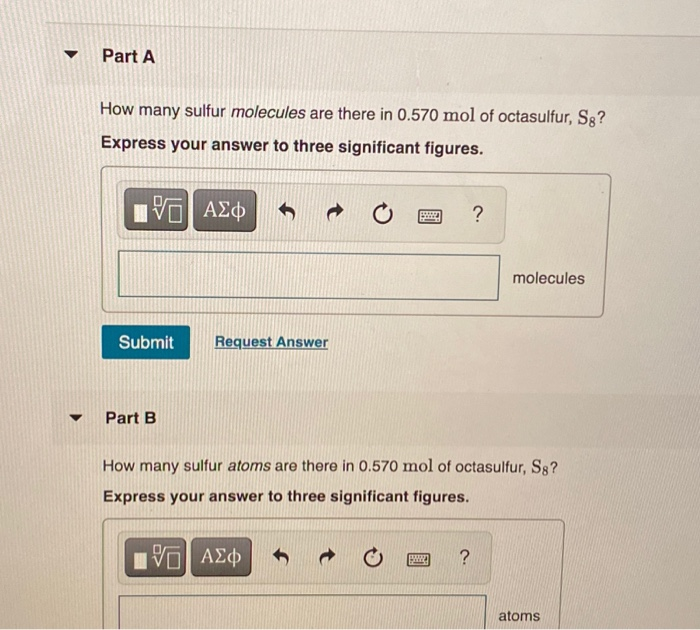Part A How many sulfur molecules are there in 0.570 mol of octasulfur, Sg? Express your answer to three significant figures. IVO AEO ? molecules Submit Request Answer Part B How many sulfur atoms are there in 0.570 mol of octasulfur, S8? Express your answer to three significant figures. ΑΣφ ? atoms

• ### If there are 0.20 moles in 8.9 grams of CO2, what is the number of CO2...

If there are 0.20 moles in 8.9 grams of CO2, what is the number of CO2 molecules? Use this equation to find the answer: Moles in sample (mol) x Avogadro's number (6.022 x 1023) = Number of sample molecules 0.20 x 1023 1.2 x 1023 8.9 x 1023 20 x 1023

• ### Be sure to answer all parts. How many moles are contained in each number of grams...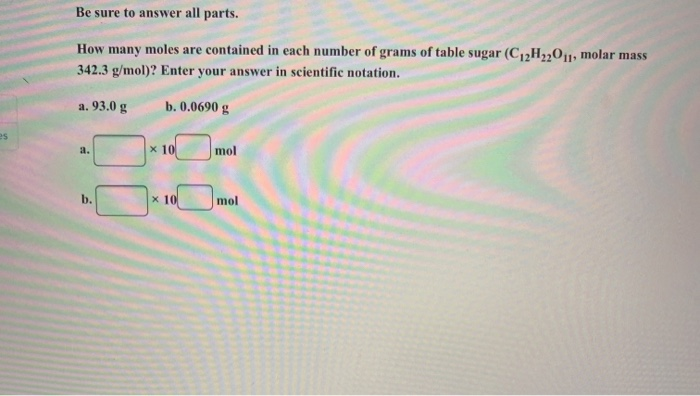Be sure to answer all parts. How many moles are contained in each number of grams of table sugar (C12H2,011, molar mass 342.3 g/mol)? Enter your answer in scientific notation. a. 93.0 g b. 0.0690 g mol

• ### Be sure to answer all parts. How many moles are contained in each number of grams...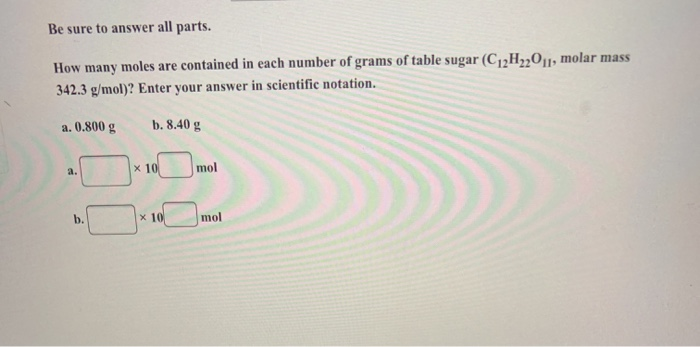Be sure to answer all parts. How many moles are contained in each number of grams of table sugar (C2H,201, molar mass 342.3 g/mol)? Enter your answer in scientific notation. a. 0.800 g b. 8.40 g mol

• ### 3) How many NH3 molecules are produced when 8.00 mol of Ca(OH)2 are completely reacted according...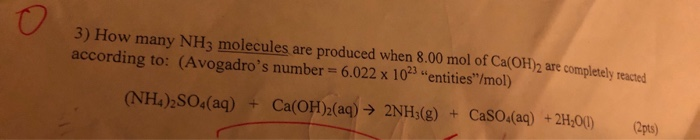3) How many NH3 molecules are produced when 8.00 mol of Ca(OH)2 are completely reacted according to: (Avogadro's number = 6.022 x 1023 entities"/mol) (NH4)2SO4(aq) + Ca(OH)2(aq) → 2NH3(g) + Caso (aq) + 2H,00) (2pts)

• ### In a 2.86E+1 g sample of NaHCO3, how many grams of sodium are present? Use two...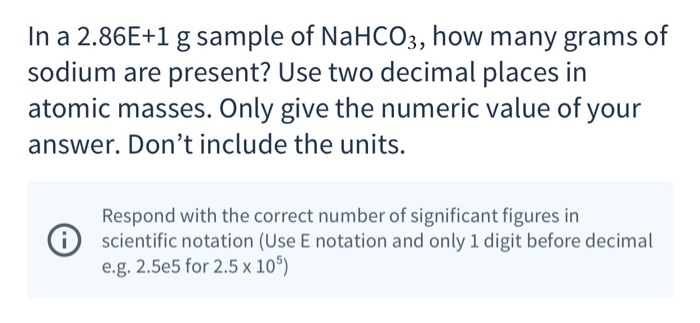In a 2.86E+1 g sample of NaHCO3, how many grams of sodium are present? Use two decimal places in atomic masses. Only give the numeric value of your answer. Don't include the units. i Respond with the correct number of significant figures in scientific notation (Use E notation and only 1 digit before decimal e.g. 2.5e5 for 2.5 x 105) In a 1.018E+2 g sample of iron(111) oxide, how many grams of iron are present? Your answer should have the...

• ### 35) What is the mass of a 0.150 mole sample of sulfur? B) 0.208 g 35)...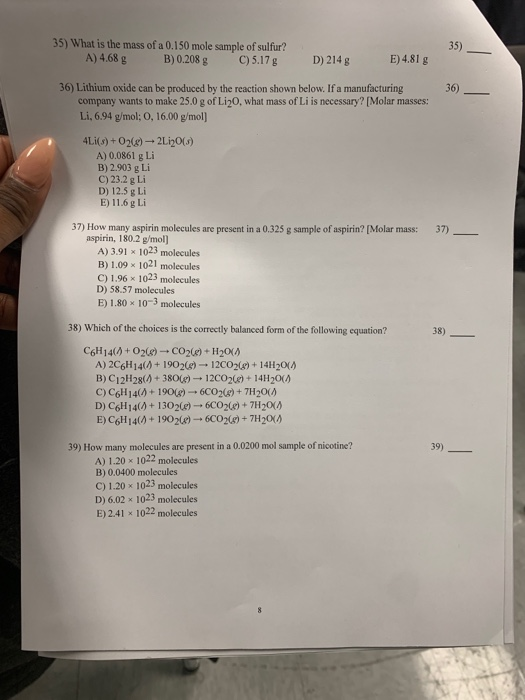35) What is the mass of a 0.150 mole sample of sulfur? B) 0.208 g 35) A) 4.68 g E)4.81 g D) 214 g C) 5.17 g 36) Lithium oxide can be produced by the reaction shown below. If a manufacturing company wants to make 25.0 g of Li20, what mass of Li is necessary? [Molar masses: Li, 6.94 g/mol; O, 16.00 g/mol] 36) 4Li(s)+O2)-2Li20(s) A) 0.0861 g Li B) 2.903 g Li C) 23.2 g Li D) 12.5 g...